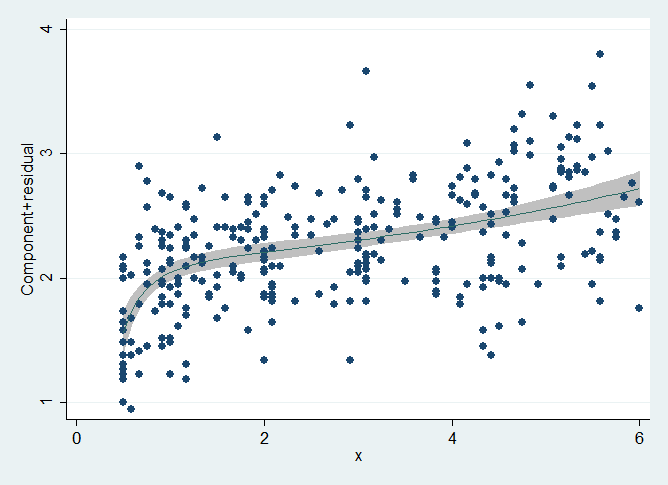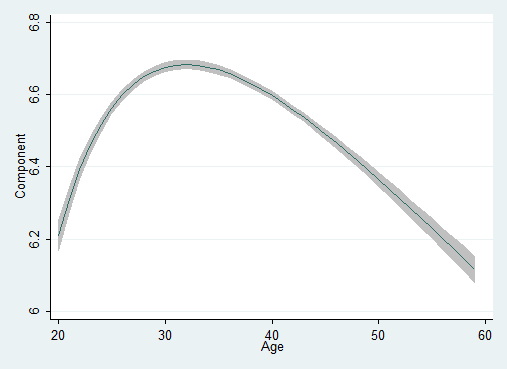»  Home »  Products »  Stata 13 »  Fractional polynomials

## Fractional polynomials

### Highlights• More flexible parameterization than with polynomials
• Prefix command for use with any estimation command

### Show me

Fractional polynomials are an alternative to regular polynomials that provide flexible parameterization for continuous variables.

For example, say we have an outcome y, a regressor x, and our research interest is in the effect of x on y. We know that y is also affected by age. One solution to this problem would be to fit a linear regression of the form

           yi = b0 + b1*xi + b2*agei + b3*agei2 + ui


An alternative would be to control for age using fractional polynomials:

           yi = b0 + b1*xi + b2*agei(p1) + b3*agei(p2) + ui


The parentheses are significant. Fractional powers are different from regular powers. For instance, age(0) is ln(age). You can see the full definition, but one example will demonstrate the power of fractional polynomials.

To fit the fractional polynomial model, we type

. fp <age>: regress y x <age>
(fitting 44 models)
(....10%....20%....30%....40%....50%....60%....70%....80%....90%....100%)

Fractional polynomial comparisons:

age     df    Deviance  Res. s.d.   Dev. dif.   P(*)   Powers

omitted      0    -83.145      0.237    625.517    0.000

linear      1   -179.287      0.232    529.376    0.000   1

m = 1      2   -295.392      0.225    413.270    0.000   3

m = 2      4   -708.663      0.203      0.000       --   -.5 -.5

(*) P = sig. level of model with m = 2 based on F with 1994
denominator dof.

Source         SS       df       MS              Number of obs =    2000

F(  3,  1996) =  347.37

Model    42.8971855     3  14.2990618           Prob > F      =  0.0000

Residual    82.1620986  1996  .041163376           R-squared     =  0.3430

Total    125.059284  1999  .062560923           Root MSE      =  .20289

y        Coef.   Std. Err.      t    P>|t|     [95% Conf. Interval]

x     .7954347   .0466225    17.06   0.000     .7040008    .8868686

age_1     11.04709   .4085977    27.04   0.000     10.24577    11.84841

age_2     13.17616   .4991682    26.40   0.000     12.19722    14.15511

_cons    -8.059393   .5469551   -14.74   0.000    -9.132056    -6.98673



We find that b1, the effect of x on y, is 0.80, but before we take that result seriously, we must ask ourselves whether we have adequately controlled for age.

. fp plot, residuals(none)Notice the shape of the y versus age curve. We could not have obtained that shape using a standard quadratic. Fractional polynomials provide a wide range of shapes that include all the shapes provided by ordinary polynomials and more. The fractional polynomial parameterization did not predetermine that the shape we obtained was skewed right. Fractional polynomials can just as easily produce skewed left shapes.

We still need to do more to convince ourselves that the curve above is adequate, but we will not do so here.

### Show me more

See the fractional polynomial manual entry.

See New in Stata 17 to learn about what was added in Stata 17.